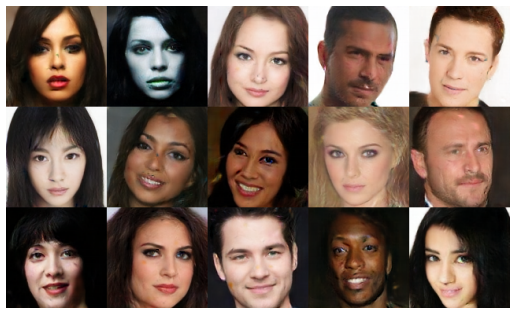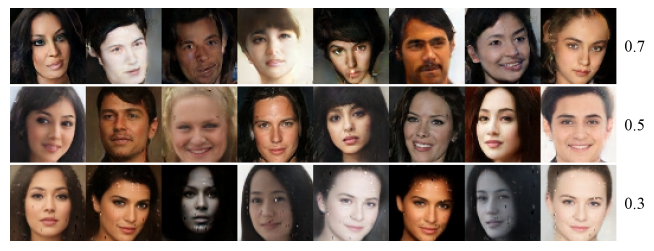# Tensorflow-BEGAN: Boundary Equilibrium Generative Adversarial Networks

I’ve covered GAN and DCGAN in past posts. In 2017, Google published a great paper. The title of paper is “BEGAN: Boundary Equilibrium Generative Adversarial Network”. “BEGAN”, what a nice name it is? Also the results are great. The generated face image looks like an image of a training dataset.BEGAN result images 128x128

#### The following contributions

• A GAN with a simple yet robust architecture, standard training procedure with fast and stable convergence.
•An equilibrium concept that balances the power of the discriminator against the generator.
• A new way to control the trade-off between image diversity and visual quality.
• An approximate measure of convergence. To our knowledge the only other published measure is from Wasserstein GAN(WGAN), which will be discussed in the next section.

In similar to EBGAN, the discriminator in BEGAN is implemented as an auto-encoder. However, the difference is that BEGAN uses Wasserstein distance for constructing the loss function. It seems to be merely a combination of EBGAN and WGAN, but it shows a surprising result. Also, networks converge more steadily than before.

### Proposed method

#### Wasserstein distance lower bound for auto-encoders

In the paper, the Wasserstein distance can be expressed as:

The above equation seems a bit difficult. But, the equation can be simplified(or bounded) as below using Jensen’s inequality:

Is there a simpler expression than 1-norm?

#### GAN objective

The GAN objective is expressed as below

Above equation is similar to that of WGAN. There are two differences

1. match distributions between losses, not between samples
2. not explicitly require the discriminator to be K-Lipschitz because Wasserstein distance are simplified.

The tensorflow code is below:

`real_loss = tf.reduce_mean(tf.abs(X - d_real))        fake_loss = tf.reduce_mean(tf.abs(g_out - d_fake))d_loss = real_loss - fake_lossg_loss = -d_loss`

We can implement Wasserstein distance using just “tf.abs”

#### Equilibrium

It is very important when adversarial training between a generator and a discriminator. To keep the balance between these models, we tune some parameters or skip training a network in the loop. A new hyper-parameter γ is introduced.

Also, γ(gamma) can control image diversity and quality.
If γ is low, we can generate high quality output but diversity of images would be decreased.At γ=0.3, diversity of images is low. There are pairs of similar image: (col 2,col 6) and (col 5, col 8)

#### Boundary Equilibrium GAN

When we put the concept of Equilibrium(γ), the objective function of BEGAN comes out below:

The default value of k0 is 0 then it’s growing bigger and bigger. D is learned well, even when the early stage G is not learned.

The tensorflow code is below:

`d_loss = real_loss - Kt * fake_loss        g_loss = fake_lossKt = Kt + lambda * (gamma * real_loss - fake_loss)`

#### Convergence measure

In BEGAN, we can get “the global measure of convergence” using equilibrium concept.

The tensorflow code is below:

`measure = real_loss + tf.abs(gamma * real_loss - fake_loss)`

Tensorflow provides a good visualization tool called tensorboard. We logged the value of convergence per each 300 steps.

`measure = real_loss + tf.abs(gamma * real_loss - fake_loss)    tf.summary.scalar('measure', measure)     merged = tf.summary.merge_all()`
`with tf.Session() as sess:    if step % 300 == 0:      summary = sess.run(merged,feed_dict={X: batch_x, Z: batch_z, Lr: learning_rate, Kt: _kt})      train_writer.add_summary(summary, epoch*total_batch+step)`

During training, we can see how the values of measure converge.

``tensorboard --logdir ./logs``

### Model architecture

The model architecture is very simple. There are no batch norm and dropout, transpose convolution, and different size of convolution kernel. Only use up/sub-sampling and 3x3 convolution and fully Connected layers.

#### Decoder

Let’s look at the above figure and write tensorflow code.

1. define fully connected layer and then reshape the output(hidden) to fit the input of convolution layer.
2. define 3x3 convolution layer with elu activation function. we repeat this job 2 times.
3. use “resize_nearest_neighbor” operator for up-sampling.
4. repeat above 2 and 3 jobs 3-times.
5. add tanh activation because we normalized the training image to [-1,1]

The code is below:

In fact, the code below creates a 64x64 image instead of 32x32. Please refer to the value of tuple in the resize and reshape function.

#### Encoder

The encoder has the reverse structure to the decoder.
The code is below:

#### Generator and Discriminator

The generator is the same structure as the decoder with only a difference in weight parameter. The discriminator is like an auto-encoder and consists of an encoder-decoder.

#### Loss function and Optimizer

We approximated the Wasserstein distance to 1-Norm. The loss functions are very simple. We minimize g_loss and d_loss using the AdamOptimizer.

### Results

You can download full tensorflow code from the below link.
https://github.com/fabulousjeong/began-tensorflow

I trained above began-networks for 10 epochs. It takes about 6 hours on Geforce 1080Ti. As learning progresses, we can see that faces are well generated. Even though I only trained about 6 hours, the result images are quite natural.

#### Reference

Berthelot et. al. — BEGAN: Boundary Equilibrium Generative Adversarial Networks, https://arxiv.org/pdf/1703.10717.pdf